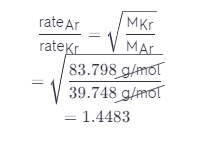# Calculate The Ratio Of Effusion Rates For Ar And Kr.

We thoroughly check each answer to a question to provide you with the most correct answers. Found a mistake? Let us know about it through the REPORT button at the bottom of the page.

Calculate the ratio of effusion rates for Ar and Kr.

Mar = 39.948 g/mol – Determine the molar mass of argon.

Mkr – 83.798 g/mol – Determine the molar mass of krypton.

Substitute these molar masses into Graham’s law to determine the ratio of effusion rates.